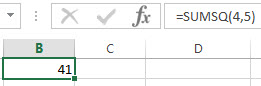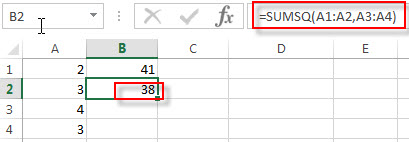## Excel SUMSQ Function

This post will guide you how to use Excel SUMSQ function with syntax and examples in Microsoft excel.

### Description

The Excel SUMSQ function returns the sum of squares of a given set of arguments.

The SUMSQ function is a build-in function in Microsoft Excel and it is categorized as a Math and Trigonometry Function.

The SUMSQ function is available in Excel 2016, Excel 2013, Excel 2010, Excel 2007, Excel 2011 for Mac.

### Syntax

The syntax of the SUMSQ function is as below:

`=SUMSQ(number1, [number2], ...)`

Where the SUMSQ function arguments are:

• number1 -This is a required argument. The number that you want the sum of the squares. You can use a single array or a reference to an array.
• [number2] -This is an optional argument. It can be up to 255 arguments in Excel 2007 and later, and it can only support up to 30 number arguments in Excel 2003.

Note:

• The arguments can be numbers or names, arrays or references that contain numbers.
• This function can count the numbers, logical values, and text representations of numbers. It means that if the text value can be interpreted as numeric values and it will be treated as Numbers. Or the function will return the #VALUE Error.
• If an argument is an array or reference, and it only count the numbers in the array or reference.
• Empty cells, logical values, text or error values in the array or reference will be ignored.

### Excel SUMSQ Function Examples

The below examples will show you how to use Excel SUMSQ Function to sum the squares of a series of values.

1# to sum of the squares of 4 and 5, enter the following formula in Cell B1.

`=SUMSQ(4,5)`2# to sum of the squares of range A1:A2 and A3:A4, enter the following formula in Cell B1.

`=SUMSQ(A1:A2,A3:A4)`### Related Functions

• Excel SUM Function
The Excel SUM function will adds all numbers in a range of cells and returns the sum of these values. You can add individual values, cell references or ranges in excel.The syntax of the SUM function is as below:= SUM(number1,[number2],…)…
• Excel SUMIF Function
The Excel SUMIF function sum the numbers in the range of cells that meet a single criteria that you specify. The syntax of the SUMIF function is as below:=SUMIF (range, criteria, [sum_range])…
• Excel SUMIFS Function
The Excel SUMIFS function sum the numbers in the range of cells that meet a single or multiple criteria that you specify. The syntax of the SUMIFS function is as below:=SUMIFS (sum_range, criteria_range1, criteria1, [criteria_range2, criteria2], …)…
• Excel SUMX2MY2 Function
The Excel SUMX2MY2 function returns the sum of the difference of squares between two supplied arrays.The syntax of the SUMX2MY2 function is as below:=SUMX2MY2 (array_x, array_y)…